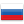### Rodionno's blog

By Rodionno, 2 months ago,I came up with a simple task, but i can't solve it faster than O(n ^ 2). So I thought that maybe someone will help me with this.

Task: given an array of numbers, you need to find segment [l, r] where value sum(l, r) * len(l, r) will be the biggest for all pairs of l, r such as 1 <= l <= r <= n. sum(l, r) = a[l] + a[l + 1] + ... + a[r], len(l, r) = r - l + 1. 1 <= n <= 10^5, -10^9 <= a[i] <= 10^9

UPD: probably it can be solved with some kind of cht or maybe li chao tree. Also it's intresting to firstly find segment with biggest sum and then try to expand it.Comments (4)
 » The answer is [1, n]
•  » » https://codeforces.com/group/YfhgFHDk6V/contest/329231/problem/DА можешь помочь с этой?
•  » » »
 » With -10^9 <= a[i] <= 10^9 constraint, it is really interesting task